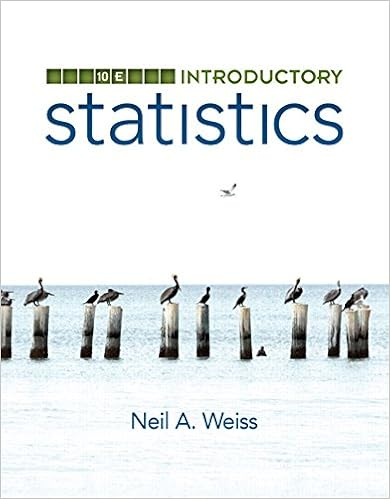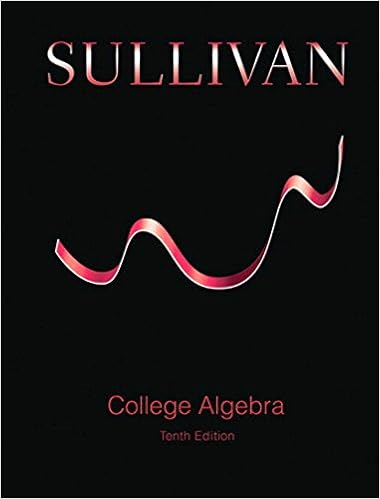# Properties of quadrilaterals properties of

• Test Prep
• 133

Course Hero uses AI to attempt to automatically extract content from documents to surface to you and others so you can study better, e.g., in search results, to enrich docs, and more. This preview shows page 101 - 104 out of 133 pages.

PROPERTIES OF QUADRILATERALSProperties of ParallelogramsIn a parallelogram,1.The parallel sides are parallel by definition.2.The opposite sides are congruent.3.The opposite angles are congruent.4.The diagonals bisect each other.5.Any pair of consecutive angles are supplementary.
##### We have textbook solutions for you!The document you are viewing contains questions related to this textbook.
Chapter 10 / Exercise 43
Mathematical Excursions
AufmannExpert VerifiedBrowse all Textbook Solutions
Properties of RectanglesIn a rectangle,1.All the properties of a parallelogram apply by definition.2.All angles are right angles.3.The diagonals are congruent.Properties of a kite1.Two disjoint pairs of consecutive sides are congruent bydefinition.2.The diagonals are perpendicular.3.One diagonal is the perpendicular bisector of the other.4.One of the diagonals bisects a pair of opposite angles.5.One pair of opposite angles are congruent.Properties of RhombusesIn a rhombus,1.Allthe properties of a parallelogram apply by definition.2.Two consecutive sides are congruent by definition.3.All sides are congruent.4.The diagonals bisect the angles.5.The diagonals are perpendicular bisectors of each other.6.The diagonals divide the rhombus into four congruent righttriangles.Properties of SquaresIn a square,1.All the properties of a rectangle apply by definition.2.All the properties of a rhombus apply by definition.3.The diagonals form four isosceles right triangles.Properties of Isosceles TrapezoidsIn an isosceles trapezoid,1.The legs are congruent by definition.2.The bases are parallel by definition.3.The lower base angles are congruent.4.The upper base angles are congruent.5.The diagonals are congruent.6.Any lower base angle is supplementary to any upper base angle.Proving That a Quadrilateral is a ParallelogramAny one of the following methods might be used to prove that a quadrilateral is a parallelogram.1.If both pairs of opposite sides of a quadrilateral are parallel, then it is a parallelogram(definition).2.If both pairs of opposite sides of a quadrilateral are congruent, then it is a parallelogram.3.If one pair of opposite sides of a quadrilateral are both parallel and congruent, then it is aparallelogram.4.If the diagonals of a quadrilateral bisect each other, then the it is a parallelogram.5.If both pairs of opposite angles of a quadrilateral are congruent, then it is a parallelogram.
Proving That a Quadrilateral is a RectangleOne can prove that a quadrilateral is a rectangle by first showing that it is a parallelogram andthen using either of the following methods to complete the proof.1.If a parallelogram contains at least one right angle, then it is a rectangle (definition).2.If the diagonals of a parallelogram are congruent, then it is a rectangle.One can also show that a quadrilateral is a rectangle without first showing that it is aparallelogram.3.If all four angles of a quadrilateral are right angles, then it is a rectangle.Proving That a Quadrilateral is a KiteTo prove that a quadrilateral is a kite, either of the following methods can be used.

Course Hero member to access this document

Course Hero member to access this document

End of preview. Want to read all 133 pages?

Course Hero member to access this document

Term
Summer
Professor
Omondi
Tags
Algebraic Expressions, Elementary arithmetic, Greatest common divisor, Divisor
##### We have textbook solutions for you!
The document you are viewing contains questions related to this textbook.The document you are viewing contains questions related to this textbook.
Chapter 10 / Exercise 43
Mathematical Excursions
AufmannExpert Verified
•••